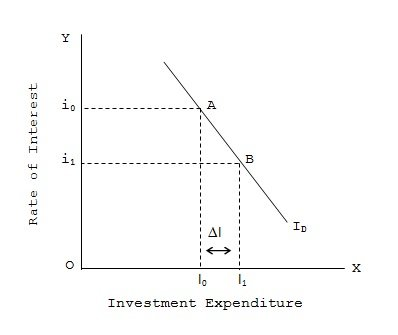Rate of Interest and Investment Expenditure

# Interest Rate and Investment Expenditure Assignment Help

Home / Macroeconomics Assignment Help / Rate of Interest and Investment Expenditure

# Rate of Interest and Investment Expenditure

The investment demand function or investment spending is negatively related to the level of rate of interest. The investment demand curve as shown is negatively sloped with respect to the rate of interest measured on the Y-axis and investment expenditure shown in the X-axis. With higher interest rate at i0 level, planned investment spending is I0. But with a fall in the interest rate i1, A rise in the rate of interest has the opposite effect on the volume of investment.### Submit Homework

Submit your homework for a free quote